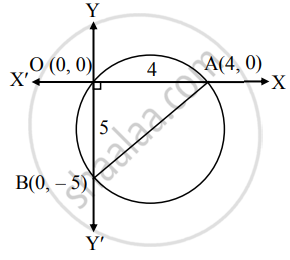# Find the equation of circle (a) passing through the origin and having intercepts 4 and −5 on the co-ordinate axes - Mathematics and Statistics

Sum

Find the equation of circle (a) passing through the origin and having intercepts 4 and −5 on the co-ordinate axes

#### SolutionSince the circle passes through the origin and having intercepts 4 and – 5 on the coordinate axes, the circle cuts the X-axis at A(4, 0) and Y-axis at B(0, – 5).

∴ ∠AOB is a right angle.

∴ seg AB is a diameter of the circle.

∴ by diameter form, the equation of the circle is

(x – 4)(x – 0) + (y – 0)(y + 5) = 0

∴ x2 – 4x + y2 + 5y = 0

∴ x2 + y2 – 4x + 5y = 0.

Is there an error in this question or solution?